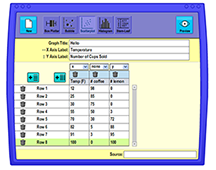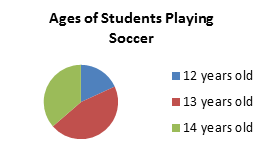Bivariate Set of Data

My Definition

Key Characteristics

The following are true of a bivariate set of data:

• Two sets of data are compared.

• Correlation describes the relationship between the two variables.

• Both variables have quantitative data.

• The relationship can be represented graphically as a scatterplot.

Example

Bivariate Set of Data Interactive

A set of sample data is included if you would like to explore creating a scatterplot with bivariate data. Click on the image below to view the sample data in a larger size.Non-example

The graph below represents univariate data.TEKS: 5(9)(B), 8(5)(C), 8(5)(D), 8(11)(A)